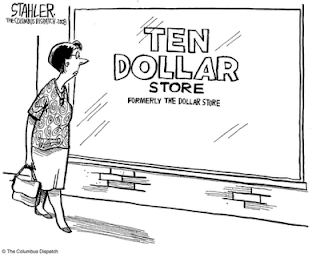## Wednesday, December 23, 2015

### Nominal vs. Real (Wages, Income)

Nominal vs. Real (wages, income)

To understand Nominal and Real we must first understand the concepts of Inflation and Purchasing Power

Inflation is an increase in the average price level of goods and services in a nation over time.(If the price of apples is increasing, and the reason is because of a flood or a drought, then this is not spoken of as inflation as the cause is specifically from a flood/draught) If the price of all goods in the country are rising then we have Inflation. (Often caused by increases in the supply of a country's currency)

Purchasing Power is the number of goods or services that can be purchased with a unit of currency.

Nominal wages = current wages

Nominal wages (Income) is the amount of money I am paid at a certain period of time.  If I'm paid \$12 an hour, then my nominal wage is \$12 dollars and hour. A nominal wage is expressed in the country's currency.  If apples cost \$1 each, then I have the ability (purchasing power) to buy 12 apples.

Time Passes, (let's say a year) and Inflation occurs, Apples have risen in prices to \$2 each.

I'm in the US and my wage is \$12 an hour, that is my nominal wage (income), and the purchasing power of my nominal wage is 6 apples at a cost of \$2 each. Due to inflation my hourly wage of \$12 has been reduced. I use to be able to purchase 12 apples for an hour's work but now I can only buy 6. My purchasing power has been reduced by 50%.

• If your income stays the same and inflation (price level rises) occurs,  then your real wage has decreases.
• If your income stays the same and instead of inflation there is deflation (price level falls), then your real wage has increased.
• If your income stays the same and there is no inflation, then your real wage is your nominal wage

Nominal Wages = Real Wages  (if there is no inflation = 0%)
If your wage (income) is \$12 an hour, and there is no inflation (price level=no change) then \$12 is your real wage.

Real Wages = Nominal Wages - Inflation
If your nominal wage is \$12 an hour and inflation is 50% then your real wage would be equal to \$6 an hour. A 50% increase in inflation will cause ones real wage to be 50% lower than the nominal wage.
So, lets look at this 2010 problem. If the workers nominal wage increased from \$10 to \$12 then the wage increased by 20%. Yet, at the same time inflation (price level) increased by 10%.

So, wages increased by 20% and inflation increased by 10%.

IF you gain 20% and inflation (eats) ten of that 20%, you are left with 10%, the answer is C.

Nominal Wages = Real Wages (Inflation = 0%)
\$10 = \$10

Real Wages = Nominal Wages - Inflation
\$9 = \$10 - \$1 (Inflation increased by 10%, this equals \$1 of a \$10 wage)

Real Wages = Nominal Wages - Inflation
\$8 = \$10 - \$2 (Inflation increased by 20%, this equals \$2 of a \$10 wage)

Real Income video - mjmfoodie